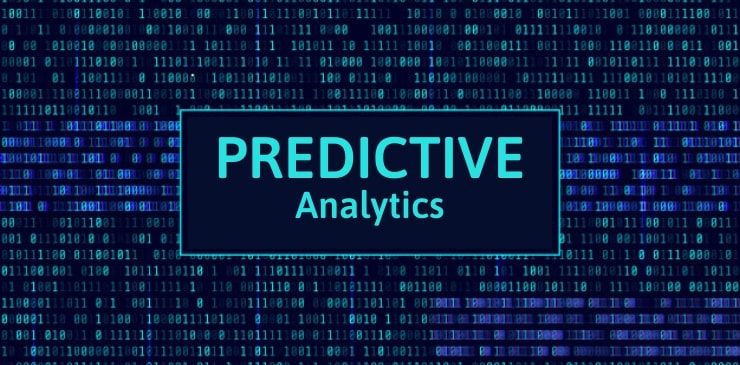What is

# What is Predictive Analytics? Definition, Techniques## Predictive Analytics

Predictive Analytics is an umbrella term to refer to the set of processes that involve applying different computational techniques in order to make predictions about the future based on the available data, statistical algorithms and machine learning techniques.

In order to train the model to predict values, Predictive analysts apply known results, with different or completely new data, in a repetitive process.

Modelling provides results in the form of predictions represented by the degree of probability of the target variable based on the significance estimated from a set of input variables.

The Predictive Analytics considers what and why surrounding critical business problems, and provides calculated predictions of what a business might expect next. Predictive Analytics points to the future and is a bit more proactive with its findings.

Predictive analytics is used to detect business opportunities, detect and reduce fraud, customer retention, predict system failures. It is also used to detect cancer in patients, the evolution of epidemics, cost savings in organizations and speech recognition.

## Predictive Modelling Techniques

1. Decision trees
2. Linear regression and logistics
3. Neural Networks
4. Bayesian analysis
5. Time series and data mining
6. K-Nearest Neighbour’s
7. Ensemble models
8. Partial least squares regression

### 1) Decision trees

They are classification models that divide the data into subsets based on categories of input variables. Decision trees are shaped like a tree in which each branch represents a choice from the number of alternatives, and each leaf represents a classification or decision.

### 2) Regression analysis

It is one of the most widely used methods in statistics. Regression analysis estimates the relationships between variables. This technique is appropriate when we can assume that continuous data follows a normal distribution, finds key patterns in large data sets. It is often used to determine the price of an asset, influence the movement of an asset.

### 3) Neural networks

Neural networks consist of sophisticated techniques capable of modelling extremely complex relationships. They have the ability to handle nonlinear relationships of the data, which makes it very interesting the more data we handle, ideal for big data analysis. Neural networks are based on pattern recognition and some “artificially intelligent” processes that allow parameters to be modelled graphically.

### 4) Bayesian analysis

The Bayesian analysis is used for computer vision and pattern recognition. In Bayesian analysis, parameters are treated as random variables and probability is defined as degrees of belief.

### 5) Time series and data mining

This method is a combination of traditional data mining techniques such as sampling, clustering and decision trees, and forecasting techniques in order to improve predictions on collected data.

### 6) K-Nearest Neighbours

K-NearestNeighbours is a non-parameterizable method of classification and regression that allows predicting values ​​of an object or the class based on training using close examples in the space of the elements.

### 7) Ensemble models

This technique is used in building a new model by training several similar models combining the results to improve precision, reduce biases, reduce variance and identify the best model to use with new data.

### 8) Partial least squares regression

Partial least squares regression is a flexible statistical technique within the regression group that can be applied to any type and form of data. It allows modeling the existing relationships between inputs and outputs even when the inputs include correlation, many outputs, and more inputs than observations.

This website uses cookies to improve your experience. We'll assume you're ok with this, but you can opt-out if you wish. Accept Read More